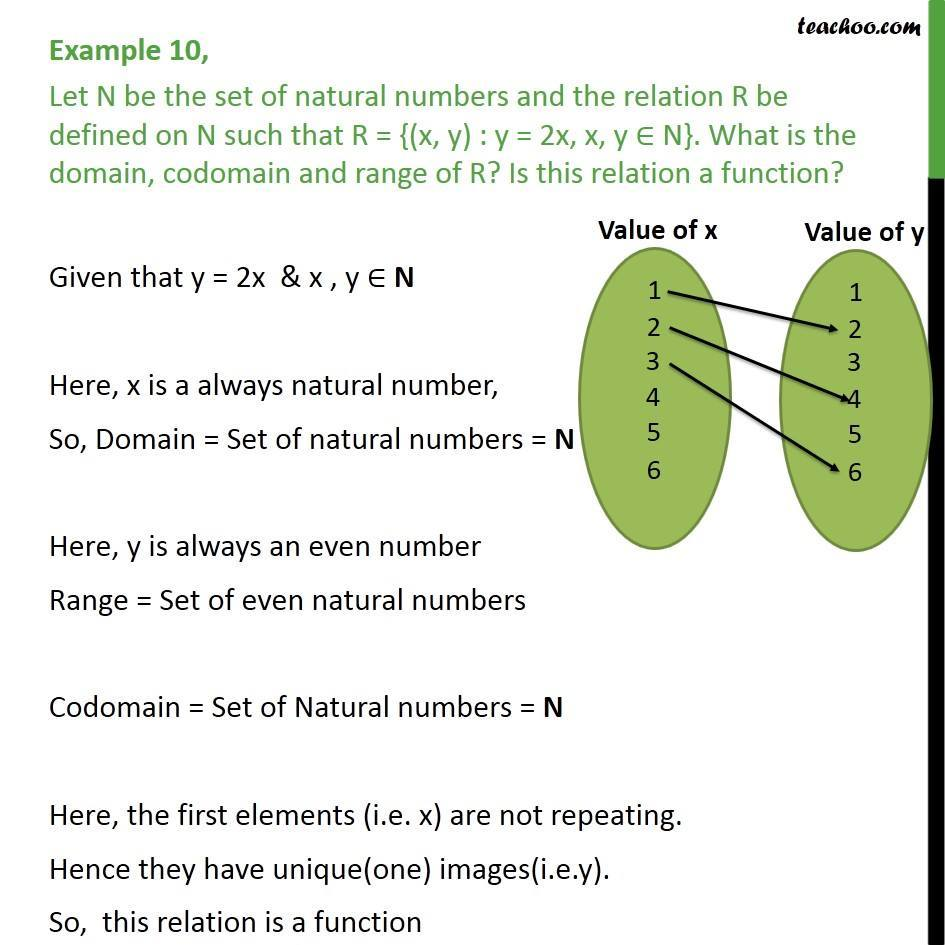1. Chapter 2 Class 11 Relations and Functions
2. Concept wise
3. Functions - Definition

Transcript

Example 10, Let N be the set of natural numbers and the relation R be defined on N such that R = {(x, y) : y = 2x, x, y N}. What is the domain, codomain and range of R? Is this relation a function? Given that y = 2x & x , y N Here, x is a always natural number, So, Domain = Set of natural numbers = N Here, y is always an even number Range = Set of even natural numbers Codomain = Set of Natural numbers = N Here, the first elements (i.e. x) are not repeating. Hence they have unique(one) images(i.e.y). So, this relation is a function

Functions - Definition